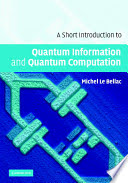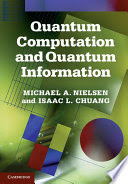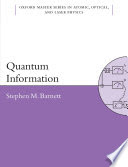### Quantum Computers

#### Course Description

Quantum mechanical principles as applied to the theory of quantum computing and quantum information will be considered. Quantum states in Hilbert space will be studied and Hermitean and unitary operators. Single qubit and multiple qubit states are introduced as well as tensor poduct of states and operators. Quantum measurement and entanglement will be described as well as quantum information and its compression and transmission. Quantum Fourier transform will be studied. Quantum algorithms will be studied. Possible realizations of quantum computers from the formal, logical and physical point of view will be given.

#### General Competencies

Students will be able to understand basic quantum mechanical principles and calculation techniques closely connected with a (quantum) information processing. They will be able to solve problems within the mathematical and physical framework of quantum information formation and its transmission. A good theoretical and practical knowledge about quantum states, entanglement principles and quantum algorithms will be given. A review of teleportation and the quantum cryptography will be given.

#### Learning Outcomes

1. Explain simple quantum systems.
2. Apply quantum mechanics to elementary processes. Explain a qubit state.
3. Explain 1/2 and 1 spin states, a linear and circular polarization and its relation to a qubit.
4. Explain a notion of an operator, Hermitean and unitary operator and Hilbert space of states.
5. Apply matrix repesentation of an operator on a different quantum mechanical situations with qubits.
6. Explain trace of an operator, eignevalues and diagonalization of an operator.
7. Relate a notion of an operator with mean value calculation in QM and pure and mixed states.
8. Explain onequbit and multiplequbit states. Explain tensor product of states and operators.
9. Explain quantum gates and quantum circuits. Explain no-cloning theorem and teleportation.
10. Apply quantum gates to quantum algorithms (Deutsch, Jozsa, Shor, Grover)

#### Forms of Teaching

Lectures

Lectures with AV support.

Exams

Midterm, homework assignments, final exam.

Exercises

Problems and examples are solved in lectures.

Consultations

Weekly consultations

Seminars

Special topics presented shortly during lectures.

E-learning

Homework assignments (Moodle)

Continuous Assessment Exam
Homeworks 0 % 20 % 0 % 20 %
Mid Term Exam: Written 0 % 40 % 0 %
Final Exam: Written 0 % 40 %
Exam: Written 0 % 80 %

#### Week by Week Schedule

1. Classical information theory. Probability theory.
2. Vector spaces. Basis. Orthogonalization.
3. Dirac bra and ket notation. Schroedinger equation. Operators. Quantum mechanical postulates. Qubits and quantum states. Multiqubit states. Tensor product of states and operators.
4. Hermitian and unitary operators. Hilbert space of states. Different bases in a vector space. Transformations.
5. Probability density operator. Quantum theory of measurement. Pure states. Mixed states. Diagonalization of an operator.
6. Pauli representation. Spin states and classical and quantum representation. Light polarization.
7. Bell's Theorem. Entangled states.
8. Midterm exam
9. Classical logic gates. Unitary transformations. Single-qubit gates. Universal gates.
10. Basic quantum circuit digrams
11. Composition and decomposition of quantum gates. No-cloning theorem.
12. Quantum algorithms. Deutsch and Deutsch-Jozsa algorithm. Quantum Fourier transform.
13. Shor algorithm. Quantum searching.
14. Review of teleportation and quantum cryptography. Possible realization of quantum computers.
15. Final exam

#### Study Programmes

Computer Engineering (profile)
Mathematics and Science (1. semester)
Computer Science (profile)
Mathematics and Science (1. semester)
Control Engineering and Automation (profile)
Mathematics and Science (1. semester)
Electrical Engineering Systems and Technologies (profile)
Mathematics and Science (1. semester)
Electrical Power Engineering (profile)
Mathematics and Science (1. semester)
Electronic and Computer Engineering (profile)
Mathematics and Science (1. semester)
Electronics (profile)
Mathematics and Science (1. semester)
Information Processing (profile)
Mathematics and Science (1. semester)
Software Engineering and Information Systems (profile)
Mathematics and Science (1. semester)
Telecommunication and Informatics (profile)
Mathematics and Science (1. semester)
Wireless Technologies (profile)
Mathematics and Science (1. semester)

#### LiteratureMichel Le Bellac (2006.), A Short Introduction to Quantum Information and Quantum Computation, Cambridge University PressMichael A. Nielsen, Isaac L. Chuang (2010.), Quantum Computation and Quantum Information, Cambridge University PressStephen Barnett (2009.), Quantum Information, Oxford University Press

#### General

ID 34566
Winter semester
4 ECTS
L3 English Level
L1 e-Learning
45 Lectures
0 Exercises
0 Laboratory exercises
0 Project laboratory